## Example Questions

1 2 3 5 Next →

### Example Question #51 : Quadratic Equations

Solve for: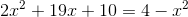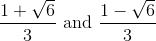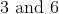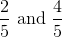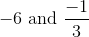Explanation:

With quadratic equations, you should always start by getting all of your terms to one side of the equation, setting this equal to:

Thus, simplifyinto: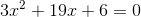Now, the next question you need to ask yourself is, "Can this be factored?" In this case, it can, though we are sometimes a bit intimidated byterms that have a coefficient like this. Factor the quadratic expression: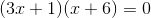If you FOIL this out, you will see your original equation.

Now, remember that you merely need to set each group equal to. This gives you the two values for: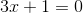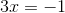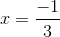For the other group, you get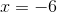.

### Example Question #41 : How To Find The Solution To A Quadratic Equation

Solve for: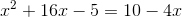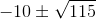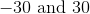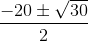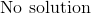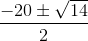Explanation:

With quadratic equations, you should always start by getting all of your terms to one side of the equation, setting this equal to:

Thus, simplifyinto: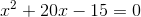Now, the next question you need to ask yourself is, "Can this be factored?" In this case, it cannot be easily factored. Therefore, you should use the quadratic formula. Recall that its general form is: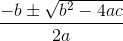For our data,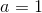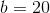, and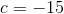.

Thus, we have: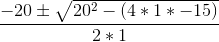Simplifying, this is: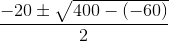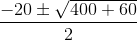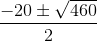Now, simplify.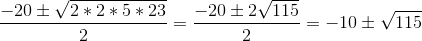### Example Question #53 : Quadratic Equations

Solve for: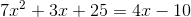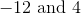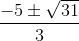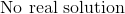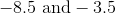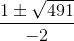Explanation:

With quadratic equations, you should always start by getting all of your terms to one side of the equation, setting this equal to:

Thus, simplifyinto: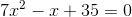Now, the next question you need to ask yourself is, "Can this be factored?" In this case, it cannot be easily factored. Therefore, you should use the quadratic formula. Recall that its general form is:For our data,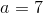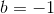, and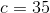.

Thus, we have: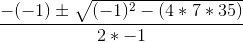Simplifying, this is: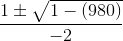Since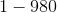is negative, you know that there is no real solution (given the problems arising from the negative square root)!

### Example Question #581 : Algebra

Solve: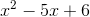andandandandandExplanation:

To solve, we must set it equal to zero. The above expression is of the form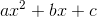, so we can use the quadratic formula: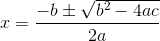to solve for the roots which areandWe can check by plugging the roots into the expression and making sure that it equals zero.

1 2 3 5 Next →

### All ACT Math Resources